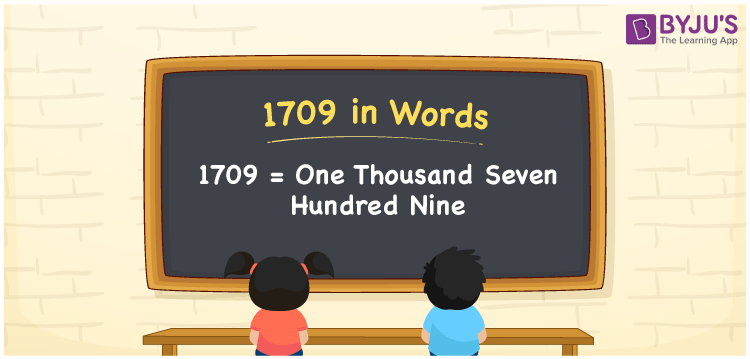# 1709 in Words

We can write 1709 in words as One thousand seven hundred nine. It is easy to convert the number 1709 into words using a place value chart. If you purchased a basket of flowers worth Rs. 1709, then you can say, “I purchased a basket of flowers for One thousand seven hundred nine rupees”. Here, One thousand seven hundred nine is the word form of the cardinal number 1709.

 1709 in words One thousand seven hundred nine One thousand seven hundred nine in Numbers 1709

## 1709 in English Words

Using the English alphabet, we can express numbers in words. So, we spell 1709 in words as “One thousand seven hundred nine”.## How to Write 1709 in Words?

Let’s understand how to write the number 1709 in words using a place value chart given below.

 Thousands Hundreds Tens Ones 1 7 0 9

Here, ones = 9, tens = 0, hundreds = 7, and thousands = 1.

Thus, we can write the expanded form of the above numbers as:

1 × Thousand + 7 × Hundred + 0 × Ten + 9 × One

= 1 × 1000 + 7 × 100 + 0 × 10 + 9 × 1

= 1000 + 700 + 9

= One thousand + Seven hundred + Nine

= One thousand seven hundred nine

Therefore, 1709 in words = One thousand seven hundred nine.

1709 is a natural number that precedes 1710 and succeeds 1708.

1709 in words – One thousand seven hundred nine

Is 1709 an even number? – No

Is 1709 an odd number? – Yes

Is 1709 a perfect square number? – No

Is 1709 a perfect cube number? – No

Is 1709 a prime number? – Yes

Is 1709 a composite number? – No

## Frequently Asked Questions on 1709 in Words

Q1

### How do you write 1709 in English words?

We can write 1709 in English words as “One thousand seven hundred nine”.
Q2

### How to write Rs. 1709 in words on a cheque?

On a cheque, we can express Rs. 1709 in words as “One thousand seven hundred nine rupees only”.
Q3

### What is the number name of 1709?

The number name of 1709 is One thousand seven hundred nine.